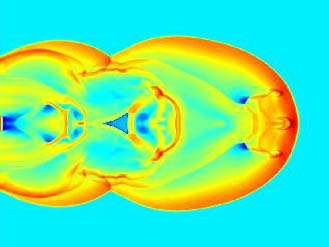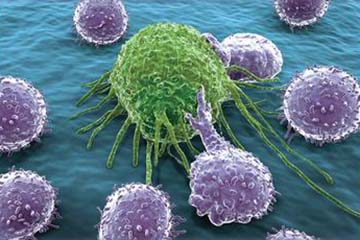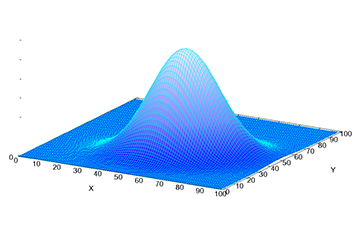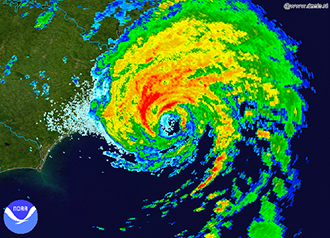# Applied Mathematics

Applied mathematics encompasses mathematical modeling and the development of computational tools often motivated by some area of application in the natural or social sciences or engineering. Our vibrant group in applied mathematics has strong interdisciplinary connections with the schools of engineering and life sciences as well as institutes such as the Biodesign Institute, the Mayo Clinic, the Global Institute of Sustainability, and the Complex Adaptive Systems Initiative.## Computational Mathematics

Development and analysis of algorithms for use in all areas of applied mathematics, science and engineering. These areas include medical imaging, atmospheric modeling, fluid dynamics, and social and biological systems modeling.## Mathematical Biology

Mathematical modeling of biological systems, including neuroscience, cancer, epidemics, and evolution.## Nonlinear Dynamical Systems

Dynamical systems describe the evolution of a state variable in time in the form of ordinary differential equations or as discrete mappings. Dynamical systems theory studies the solutions of such equations and mappings and their dependence on  initial conditions and  parameters. Research in nonlinear dynamical systems  in particular is interested in qualitative changes of the solution type as parameters are changed (bifurcations) and in chaotic behavior of solutions. Applications include atmospheric science, the behavior of fluids, social and biological systems.## Probability

Probability is the study of random phenomenon and the likelihood of random events occurring. An example of a random event includes the outcome of flipping a coin, the uncertain path of a hurricane, or an unexpected epidemic. Probability uses theoretical mathematical tools and has numerous applications in science, engineering and finance.## Actuarial Science

The analysis of risk in finance and insurance.

## Systems and Control Theory

The study of time-dependent systems of equations with feedback inputs to modify output; examples and applications include the cruise control on an automobile and automatic pilot systems on aircraft.